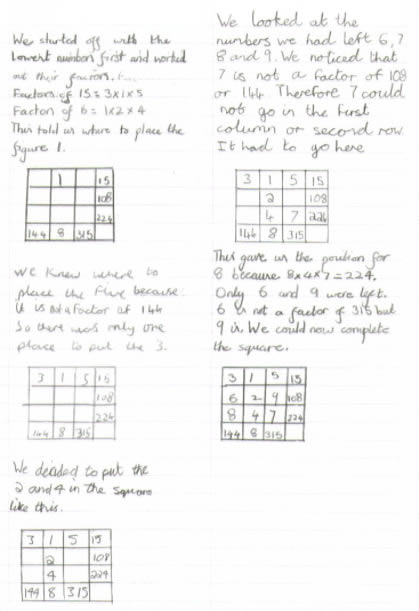#### You may also like### Pebbles

Place four pebbles on the sand in the form of a square. Keep adding as few pebbles as necessary to double the area. How many extra pebbles are added each time?### Bracelets

Investigate the different shaped bracelets you could make from 18 different spherical beads. How do they compare if you use 24 beads?### Sweets in a Box

How many different shaped boxes can you design for 36 sweets in one layer? Can you arrange the sweets so that no sweets of the same colour are next to each other in any direction?

# Multiplication Squares

##### Age 7 to 11Challenge Level

This solution came from Adam from Moorfield Junior School.

This is how I arranged the numbers from one to nine in a multiplication square once and once only:

 1st row: 3x1x5=15 2nd row: 6x2x9=108 3rd row: 8x4x7=224 1st column: 3x6x8=144 2nd column: 1x2x4=8 3rd column: 5x9x7=315

He is right but doesn't tell us how he worked it out. This solution is from James, Terry, John and Indiya from Sabden Primary School, Clitheroe, Lancashire.This solution is from Ailsa from Romsey Abbey Primary School, Romsey, Hampshire. She says:

I managed to crack the Multiplication Square, below, here is how I did it:

 3 1 5 15 6 2 9 108 8 4 7 224 144 8 315

First I worked out that the only way to make 15 was to use the numbers 1, 3 and 5. I then discovered that the only way to make 8 was to use the numbers 1, 2 and 4. Therefore the 1 had to go in the top row and second column. I then worked out 5 had to go in the top row and third column because 315 is a multiple of 5 and 144 is not, so that meant that the 3 had to go in the top row in the first column.

Then I divided 108 by 2, the answer was 54, and then made 54 using the numbers I had left, 6, 7, 8 and 9, the answer was 9 x 6, so I knew that 9 and 6 were the 2 other numbers that went in the second row. 6 is not a multiple of 315 so 6 went in the first column and 9 went in the third column.

So now the only 2 numbers left were 7 and 8 so I worked the answer out by discovering how to make 315. I multiplied 5 by 9 to give me the answer 45 and then tried multiplying it by both 7 and 8. 7 was the correct number so 7 went in the third column and 8 went in the first.

This is a really clear description of your method, well done!

Kaden from Coleridge Community College, Cambridge also sent a correct solution. Well done!

We have had some other solutions, from a different school, that had got the 6 and the 9 swapped over. Will it work then?